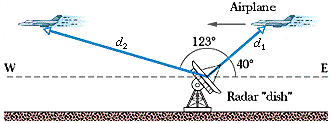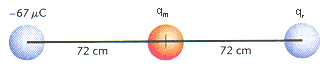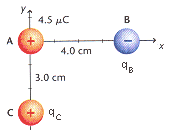May
23

## Access to protected areas of this website

To access any protected areas of this website with physics content please look at the right-hand side of the graphic at the top of the page.  For students or parents wishing to access copyrighted UIL materials please contact me for your credentials.

Why have I done this?

In an effort to continue to make my content freely available to my students, parents, colleagues and other educators, but to keep “web-bots” from stealing content from this domain, I have embedded the access information into the graphic at the top of the webpage.  That information should open all publicly accessible portions of this site. If you have any problems accessing course content or if the password does not appear to work, please contact me as soon as possible.

Feb
18

Feb
11

## HW 2.2 Item #4

Several students asked about this problem after class last night.  Let’s take a look: (using my values from Webassign)

A radar station detects an airplane approaching directly from the east. At first observation, the range to the plane is d1 =390 m at 40° above the horizon. The airplane is tracked for another 123° in the vertical eastwest plane, the range at final contact being d2 = 770 m. See Fig. 4-30. Find the displacement of the airplane during the period of observation.My first thoughts on the problem were “Oh, its just the side of a triangle defined by d1 and d2 as the other two sides.  I can probably just use trig to figure it out geometrically.”  Well, this is true, but in order to work it out as a simple triangle, we would need the key piece of information that the plane is flying horizontally, with no change in elevation.  Unfortunately this is NOT true, as a closer look at the values will tell us.  We will get to that in a moment.  What we actually need to do is define displacement faithfully as the change in position: final position – initial position.

First, let’s analyze the positions determined by the radar:

Make a right triangle out of each vector and find the horizontal and vertical components.

d1x: 390 cos(40) = 299 m
d1y: 390 sin(40) = 251 m

d2x: 770 cos(17) = 736 m
d2y: 770 sin(17) = 225 m

Now find the change in the horizontal and vertical positions independently of each other:

Deltax = -736 – 299 = -1035 m
Deltay = 225-299 = -74 m  (clearly not the same height, so not flying horizontally as mentioned above)

Magnitude of displacement = sqrt (1035^2 + 74^2) = 1038 m
Direction of displacement = arctan (74/1035) = 4 degrees S of W, or 184 degrees

Now, there does seem to be a problem with the webassign answer on part b for some of your assignments, so I will throw out that part.  It does not seem to be coded correctly, but I can’t locate the error.  Sorry for any confusion.

Feb
07

## Linear Motion 1.2 #4, airplane over a slope

A high-performance jet plane, practicing radar avoidance maneuvers, is in horizontal flight of h = 45 m above the level ground. Suddenly, the plane encounters terrain that slopes gently upward at 4.3°, an amount difficult to detect (see Fig. 2-16). How much time does the pilot have to make a correction to avoid flying into the ground? The speed of the plane is1300 km/h.

You will have different numbers than mine for this problem, but the principle of solving it is the same.  First, let’s consider the scenario.  Is the plane changing it’s speed?

If you answered “No” you win a prize.  Since there is no acceleration in the scenario, the actual physics work is very simple.  We will be playing with the defn. of a constant velocity:

v=(delta)x/t         and we will be solving for t, as per the problem.

The rest of the problem is just geometry and dimensional analysis.

For the geometry:

Consider the slope as a right triangle, the height of which is defined by the height that the plane is flying.  The angle opposite that leg is the 4.3 degree slope (from my problem) and we have an unknown hypotenuse, and unknown adjacent leg.  The adjacent leg is the key.  If the plane flies that far, it will crash into the hill.  So we need to find the length of that leg.

Using tangent ooperator:

tan 4.3 = 45/adj

adj = 45/tan 4.3 = 598.5 m

Now we know how far the plane can fly before it runs into the hill.

We also know the speed, but we must convert to m/s.  My conversion results in a velocity of 361.1 m/s

Now the physics:

v=(delta)x/t

t = (delta)x/v = 598.5 / 361.1 = 1.66 s

Jan
22

## Gen Phys 1: First class assignment and webassign information

Don’t forget to set up your webassign account.  The instructions I gave you in class can be found here.

Frequently asked questions regarding webassign and online homework:
WebAssign-FAQ-UD

There may be a glitch in assignment 1.2, problem 13.  If you encounter an issue, please email me.

Finally, I realized that I accidentally copied an older and somewhat less clear version of the “Mechanics Discovery” assignment at the front of your practice packet that I asked you to do for next class.  The content is generally the same, but the instructions are slightly less clear than the version linked in the unit page section of the website which you can find HERE.  Below, please find solutions worked out for that introductory assignment.
Mechanics Discovery–solutions

Also on the unit page section of the website, you will find the calculus notes from the first class, plus some practice problems involving some basic derivatives and integrals.  If you have never taken any calculus before this semester, or if you wish to brush up, you might want to do this practice.  We will being applying predominately power-rule derivatives and integrals as we dive into kinematics in our next classes.

Looking forward to our next meeting!

Apr
01

## PreAP: Electrostatics 1 HW helps

Several folks have inquired about HW questions, particularly #’s 19-22.  These are certainly the more difficult problems on the HW assignment.  I will provide you with both some general information and some specific helps on each.

First, 19 & 20 are essentially the same.  19 has a North-South orientation, while 20 has an East-West orientation.  21 & 22 are similar except that you have more complicated 2-D vectors to consider.  Secondly, and perhaps the most important help I can give you: Ignore the signs when you use Coulomb’s Law.  Ignore the signs while you are doing your calculations and when you are done ask yourself, “What is the direction of this force?”  The remainder of the problem will depend on how you utilize that info.

Some background: If you keep the signs in the calculation when doing Coulomb’s Law Q1*Q2 give some interesting results.  If you have like charges (either + &+ or – & -) you will always end up with a positive force.  What does this mean?  Well, like charges repel, so a positive result must mean the charges push each other away.  Does the opposite hold?  Well if Q1 & Q2 have opposite signs (e.g., + & -) and you apply Coulomb’s Law your result will be negative.  This means an attractive force, or a force along a direction toward the second charge.

Let’s begin with the easier scenarios (19 & 20).

Three particles are placed in a line. The left particle has a charge of -67 µC, the middle, qm = +42 µC, and the right, qr = –87 µC. The middle particle is 72 cm from each of the others, as shown in Figure 20-13.Figure 20-13.

(a) Find the net force on the middle particle.
Let’s get some labels here.  Call the Left particle A, middle B, and right C.

In order to find the force on B we only need to calculate the force of A on B and of C on B.  The force of A on C is irrelevant to this problem.

So Fnet = FAB + FCB

FAB = ?    B is pulled to the LEFT

FCB = ?     B is pulled to the RIGHT

Since these forces are in opposite directions you need to treat them so, so when you calculate the net or total force you must SUBTRACT.

Part B)

Essentially the same idea, but a little different.  TO find the force on the right particle, you want

Fnet = FAC + FBC.

You already know the value of FBC from part A, but you must reconsider that the direction will be to the LEFT, relative to charge C.

FAC will need to be calculated.  It’s direction is repulsive, so to the RIGHT on charge C.  Again, subtraction will be called for.  A final caution: When finding the magnitude of FAC, be sure to consider the whole distance between particles A and C.

#’s 21 & 22:

We will look at number 21.  Like 19 and 20 you have a couple forces to calculate and consider.  The only difference here is that you must apply your rules for adding vectors at funky angles (find the x- and y-components for each vector, add them, find the resultant).  As always, one must consider direction whenever we “add” vectors.  Looking closely at one of your classmates versions of 21:

Three charged spheres are located at the positions shown in Figure 20-14. Find the total force on sphere B. (qB = -7.4 µC, and qC = +5.5 µC.)First use Coulomb’s Law to find the two forces: The force of A on B, and the force of C on B.  Both are attractive, so charge B is pulled generally toward the left.
When I solved with your numbers, I found the following vectors:
187 N to the Left
146 N @ 36.9 degrees South of West

Breaking into components and adding the x- and y-directions respectively I get:
X: -304 (left)
Y: -87.7 (South)

Using trig an Pythagorean, I conclude with: 316 N @ 16.1 degrees South of West (take care in putting that into Webassign as you need to refernce the whole angle from 0-degrees).

Mar
30

## AP-C: Magnetic field practice from SMU

Loads of Practice problems:

http://www.physics.smu.edu/vega/p1308/Homework%20Chapters/chap_30.pdf

Mar
30

## AP-C: B-fields old quiz for practice (With KEY)

Below is a quiz that I have assigned as a take home quiz several times in recent years.  It focuses on the B-force only, so more the first half of our most recent unit.  Solutions are worked out in the pdf document.

Quiz 19.2–AP–Magnetic Fields

B-fields quiz 1–KEY

Mar
27